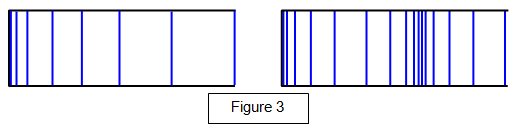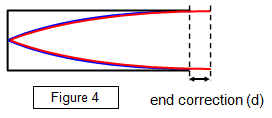# The Physics of sound in tubes

This part of the Physics of sound is the basis of all wind instruments, from the piccolo to the organ. Basically the ideas are very simple but they can become complex for a specific musical instrument. For that reason we will confine ourselves to a general treatment of the production of a note from a uniform tube.The stationary waves set up by the vibrations of the air molecules within the tube are due to the sum of two travelling waves moving down the tube in opposite directions. One of these is the initial wave and the other its reflection from the end of the tube.

All air-filled tubes have a resonant frequency and if the air inside them can be made to oscillate they will give out a note at this frequency. This is known as the fundamental frequency or first harmonic.

Higher harmonics or overtones may also be obtained and it is the presence of these harmonics that gives each instrument its individual quality. A note played on a flute will be quite unlike one of exactly the same pitch played on a bassoon!

A harmonic is a note whose frequency is an integral multiple of the particular tube's or string's fundamental frequency.

Tubes in musical instruments are of two types:
(a) open at both ends, or
(b) open at one end and closed at the other.

The vibration of the air columns of these types of tube in their fundamental mode are shown in Figure 1. Notice that the tubes have areas of no vibration or nodes at their closed ends and areas of maximum vibration or antinodes at their open ends.

An antinode also occurs at the centre of a tube closed at both ends in this mode.

Nodes are areas where the velocity of the molecules is effectively zero but where there is a maximum variation in pressure, while the reverse is true for antinodes.

Some of the higher harmonics for the different tubes are shown in Figure 2. Notice that a closed tube gives odd-numbered harmonics only, while the open tube will give both odd and even-numbered.Notice that although the sound waves in the tubes are longitudinal it is conventional to represent them as transverse vibrations for simplicity. However two examples of what are really going on is given for completeness.North Curry village church organ

If the velocity of sound is denoted by v and the length of a tube by L, then for a tube closed at one end the fundamental frequency is given by:

Fundamental frequency (f) = v/wavelength = v/4L

For a tube open at both ends the fundamental frequency is given by:

Fundamental frequency (f) = v/wavelength = v/2L

### End correctionsThe vibrations within the tube will be transmitted to the air just outside the tube, and the air will then also vibrate. In accurate work we must also allow for this effect, by making an end correction (Figure 4).

This means that we consider that the tube is effectively longer than its measured length by an amount d, that is:

The true length = L ± d. The equation for a closed tube then becomes:

f = v/4[L+d]

## Measurement of the velocity of soundThe velocity of sound in air may be found quite simply by using the resonance of a column of air in a tube. An open- ended tube is placed in a glass cylinder containing water, as shown in Figure 4, so that the water closes the bottom end of the tube. A tuning fork of known frequency is sounded over the upper end, the air in the tube vibrates and a note is heard. The length of the air column is adjusted by raising the tube out of the water until a point is found where resonance occurs and a loud note is produced. At this point the frequency of the tuning fork is equal to the resonant frequency of the tube.
In its fundamental mode the wavelength A is four times the length of the air column (L), that is: l= 4L
Since velocity = frequency x wavelength the velocity of sound may be found. For accurate determinations the following precautions should be taken:
(a) the temperature of the air should be taken, since the velocity of sound is temperature-dependent, and
(b) the end correction should be allowed for. This may be done by finding the resonance for the second harmonic with the same tuning fork.

Example problems
1. A 1.5 m tube is open at both ends. What is:
(a) the frequency of the fundamental (first harmonic)
(b) the wavelength of the first overtone (second harmonic)
Take the speed of sound in the air in the tube to be 330 ms-1.
(a) Length of the tube = 0.5 x wavelength = 1.5 m Wavelength = 3 m
Fundamental frequency = v/λ = 330/3 = 110 Hz
(b) Length of tube = Wavelength of second harmonic = 1.5 m 2. A 1.2 m long air filled tube, closed at one end has a resonant frequency of 70 Hz. Calculate the speed of sound in the air.
Wavelength = 4 x length of tube = 4.8 m
Speed of sound = 70 x 4.8 = 336 ms-1